# showShape

Display shapes on image, video, or point cloud

## Syntax

``showShape(shape,position)``
``showShape(___,Name=Value)``

## Description

example

````showShape(shape,position)` displays one or more instances of a shape `shape` in the current axes at the specified locations `position`.```

example

````showShape(___,Name=Value)` specifies options using one or more name-value arguments in addition to the previous syntax. For example, ` showShape("rectangle",bboxes,Color="yellow")` sets the color for the shapes to yellow.```

## Examples

collapse all

Read an image into the workspace.

`I = imread("visionteam1.jpg");`

Create an aggregate channel features (ACF) people detector.

`detector = peopleDetectorACF()`
```detector = acfObjectDetector with properties: ModelName: 'inria-100x41' ObjectTrainingSize: [100 41] NumWeakLearners: 2048 ```

Detect people in the image.

`[bboxes,scores] = detect(detector,I);`

Display the image with a labeled rectangle. Display the associated detection score around each detected person.

```figure imshow(I) labels = "person:"+scores; showShape("rectangle",bboxes,Label=labels)```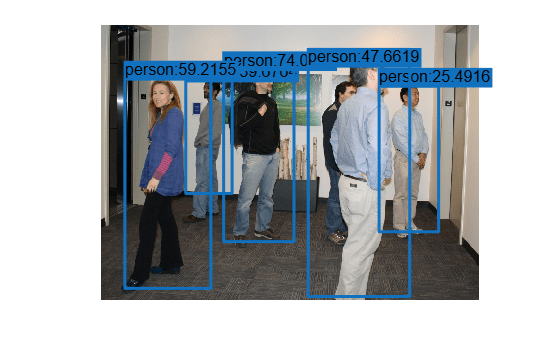Read point cloud data into the workspace.

`ptCloud = pcread("teapot.ply");`

Display the point cloud data.

```figure pcshow(ptCloud) xlabel("X") ylabel("Y") zlabel("Z")```

Define a cuboid and display it in green with an opacity of `0.5`.

```pos = [0.3753 0 1.65 6 4 3 0 0 0]; showShape("cuboid",pos,Color="green",Opacity=0.5)```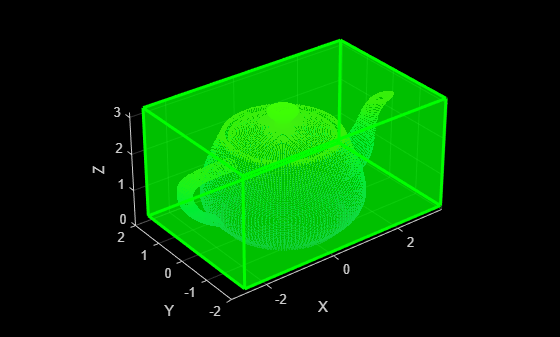Read point cloud data into the workspace.

`ptCloud = pcread("teapot.ply");`

Define a rotation matrix and 3-D transform to rotate the point cloud, and an associated cuboid, by 5 degrees.

```rot = 5; R = [ cosd(rot) sind(rot) 0 0; ... -sind(rot) cosd(rot) 0 0; ... 0 0 1 0; ... 0 0 0 1]; tform = affine3d(R);```

Compute the x and y rendered limits of the point cloud to ensure that the point cloud is not clipped during rotation.

```pcLimits = abs([ptCloud.XLimits ptCloud.YLimits]); maxLimit = max(pcLimits);```

Add an additional margin to the plot to prevent the cuboid from being clipped during rotation.

```margin = 1; maxLimit = maxLimit + margin; xlimits = [-maxLimit maxLimit]; ylimits = [-maxLimit maxLimit]; zlimits = ptCloud.ZLimits;```

Create a player for visualizing the point cloud.

`player = pcplayer(xlimits,ylimits,zlimits);`

Customize the player axis labels.

```xlabel(player.Axes,"X (m)"); ylabel(player.Axes,"Y (m)"); zlabel(player.Axes,"Z (m)");```

Define a cuboid around the point cloud.

`cuboidPosition = [0.3753 0 1.65 6 4 3 0 0 0];`

Define the output view for a cuboid rotation. Use the same limits as the player so that the cuboid is not clipped. Then, display the rotated point cloud and cuboid.

```gridSize = [1 1 1]; ref = imref3d(gridSize,xlimits,ylimits,zlimits); for i = 1:round((360/rot)) % Rotate point cloud. ptCloud = pctransform(ptCloud,tform); % Rotate the cuboid. cuboidPosition = bboxwarp(cuboidPosition,tform,ref); % Show rotated point cloud data. view(player,ptCloud) % Show rotated cuboid. showShape("cuboid",cuboidPosition, ... Parent=player.Axes, ... Color="green", ... Opacity=0.5) % Use drawnow to synchronize point cloud and shape visualization. drawnow end```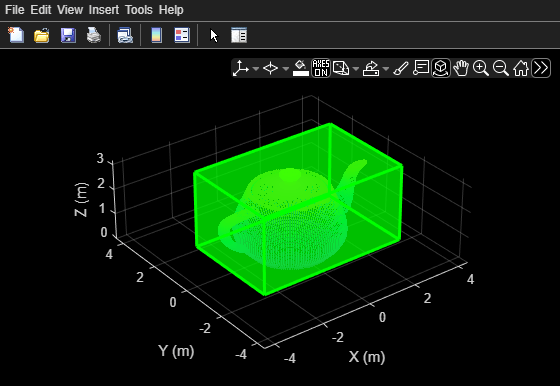## Input Arguments

collapse all

Type of shape, specified as `"rectangle"`, `"filled-rectangle"`, `"line"`, `"polygon"`, `"filled-polygon"`, `"circle"`, or `"filled-circle"`, `"projected-cuboid"`.

Data Types: `char`

Positions and sizes of shapes, specified according to the type of shapes, described in this table.

Bounding BoxDescription
`rectangle`

Defined in spatial coordinates as an M-by-4 numeric matrix with rows of the form [x y w h], where:

• M is the number of axis-aligned rectangles.

• x and `y` specify the upper-left corner of the rectangle.

• w specifies the width of the rectangle, which is its length along the x-axis.

• h specifies the height of the rectangle, which is its length along the y-axis.

`rotated-rectangle`

Defined in spatial coordinates as an M-by-5 numeric matrix with rows of the form [xctr yctr xlen ylen yaw], where:

• M is the number of rotated rectangles.

• xctr and yctr specify the center of the rectangle.

• xlen specifies the width of the rectangle, which is its length along the x-axis before rotation.

• ylen specifies the height of the rectangle, which is its length along the y-axis before rotation.

• yaw specifies the rotation angle in degrees. The rotation is clockwise-positive around the center of the bounding box.`cuboid`

Defined in spatial coordinates as an M-by-9 numeric matrix with rows of the form [xctr yctr zctr xlen ylen zlen xrot yrot zrot], where:

• M is the number of cuboids.

• xctr, yctr, and zctr specify the center of the cuboid.

• xlen, ylen, and zlen specify the length of the cuboid along the x-axis, y-axis, and z-axis, respectively, before rotation.

• xrot, yrot, and zrot specify the rotation angles of the cuboid around the x-axis, y-axis, and z-axis, respectively. The xrot, yrot, and zrot rotation angles are in degrees about the cuboid center. Each rotation is clockwise-positive with respect to the positive direction of the associated spatial axis. The function computes rotation matrices assuming `ZYX` order Euler angles [xrot yrot zrot].

The figure shows how these values determine the position of a cuboid.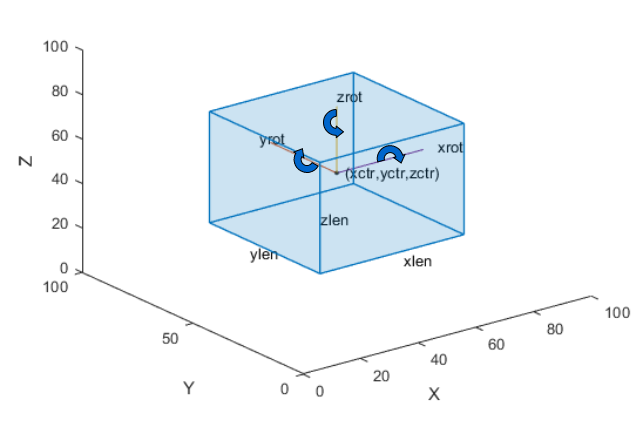`circle`

Defined in spatial coordinates as an M-by-3 numeric matrix with rows of the form [xctr yctr radius], where:

• M is the number of circles.

• xctr and yctr specify the center of the circle.

`line`

Defined in spatial coordinates as a P-by-2 matrix in which each row is an [x y] endpoint or a 1-by-2P vector of consecutive endpoints of the from [x1 y1 x2 y2xP xP].

• P is the number of endpoints.

• [x1 y1], [x2 y2], and xP xP describe endpoints.

To specify several lines that contain different numbers of endpoints, you can use the cell array format described for a polygon.

`polygon`

Defined in spatial coordinates as an M-by-1 cell array in which each cell contains an L-by-2 matrix of [x y] vertex locations or a 1-by-2L vector of consecutive vertex locations of the form [x1, y1, x2,y2, … xL,yL ], where:

• M is the number of polygons.

• [x1 y1], [x2 y2] describe vertex locations or endpoints.

• L is the number of vertices in a polygon or endpoints in a line. Each polygon or line can have different number of vertices or endpoints.

` projected-cuboid`

An 8-by-2-by-M array or an M-by-8 matrix, where M specifies a projected cuboid.

When specified as an 8-by-2-M array, each row must contain the $\left[\begin{array}{cc}x& y\end{array}\right]$ location of a projected cuboid vertex. The vertices are connected to form a cuboid with six faces. The order of the input vertices must match the order shown in the diagram.

When specified as an M-by-8 matrix, each row specifies the front-facing and rear-facing sides of a projected cuboid in the form,

`$\left[\begin{array}{cccccccc}x1& y1& w1& h1& x2& y2& w2& h2\end{array}\right]$`

where, [x1 y1] and [x2 y2] specify the upper-left coordinates of the front-facing and back-facing sides, respectively. [w1 h1] and [w2 h2] specify the corresponding width and height.### Name-Value Arguments

Specify optional pairs of arguments as `Name1=Value1,...,NameN=ValueN`, where `Name` is the argument name and `Value` is the corresponding value. Name-value arguments must appear after other arguments, but the order of the pairs does not matter.

Before R2021a, use commas to separate each name and value, and enclose `Name` in quotes.

Example: ` showShape("rectangle",bboxes,Color="yellow")` sets the color for the shapes to yellow.

Shape label, specified as a scalar, M-element vector, or an M-element cell array of character vector, where M specifies the number of shapes.

If the input is a scalar value, then the function applies the label to each shape. If the input is an M-element vector or cell array, then the function applies the unique label to the corresponding shape, using the order in which the shapes are specified to the function.

Shape color, specified as one or more RGB triplets, or one or more (MATLAB `ColorSpec`) color or short color names. Color values must be specified in the range `[0,255]`. Values that have a range of `[0,1]` must be scaled by a value of 255 before using it with this function. For example, `[255 255 255].*colorvalue`

ColorFormatExample
Specify one color for all shapes

Color name

`"r"`

`"red"`

RGB triplet

`[255 0 0]`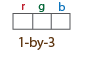Specify a color for each shapeM-element vector

`["red","yellow","blue"]`

M-by-3 matrix, as a list of RGB values

```255 0 0 255 0 0 0 255 255```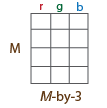Opacity of the shape fill, specified as an M-element vector of numeric values in the range [`0 1`], where M specifies the number of shapes. To use the same opacity for all shapes, specify a scalar opacity value. For a fully opaque shape fill, set `Opacity` to `1`.

Output axes, specified as an Axes Properties graphics object.

Border line color, specified as one or more RGB triplets, or one or more color or short color names. To use the same color for all border lines, specify a single (MATLAB `ColorSpec`) color name or a single RGB triplet.

To use a different color for each shape, specify an M-element vector or cell array of color names or an M-by-3 numeric matrix in which each row is an RGB triplet. M is the number of shapes specified to the function.

Border line width in pixels, specified as a positive scalar integer or an M-element vector of positive scalar integers, where M is the number of shapes. To use the same line width for all shapes, specify a positive scalar integer. Otherwise, specify an M-element vector of positive integers.

Border line opacity of the shape fill, specified as an M-element vector of numeric values in the range [```0 1```], where M is the number of shapes. To use the same opacity for the border line of all shapes, specify a scalar opacity value. For a fully opaque border line, set `Opacity` to `1`.

Label text color, specified as one or more RGB triplets, or one or more color names or short color names. To use the same color for the text of all labels, specify a single (MATLAB `ColorSpec`) color name or a single RGB triplet.

To use a different color for text of each label, specify an M-element vector or cell array of color names or an M-by-3 numeric matrix in which each row is an RGB triplet. M is the number of labels specified to the function.

Label opacity, specified as an M-element vector of numeric values in the range [`0 1`], where M is the number of shapes. To use the same opacity for all labels, specify a scalar opacity value. For a fully opaque label, set `Opacity` to `1`.

Label font, specified as `"FixedWidth"` or a font that your system supports. To display and print text properly, you must select a font that your system supports. If you select an unsupported font, the function returns an error. If you specify `"FixedWidth"`, the function uses the font specified by the `FixedWidthFont` property of the Root Properties object. The fixed-width font relies on the value of `get(0,'FixedWidthFontName')` .

Label font size in point units, specified as a positive scalar.

## Version History

Introduced in R2020b

expand all